# python数据分析：流量数据化运营（中）——流量数据波动原因下探分析

## 从细分到多层下钻数据分析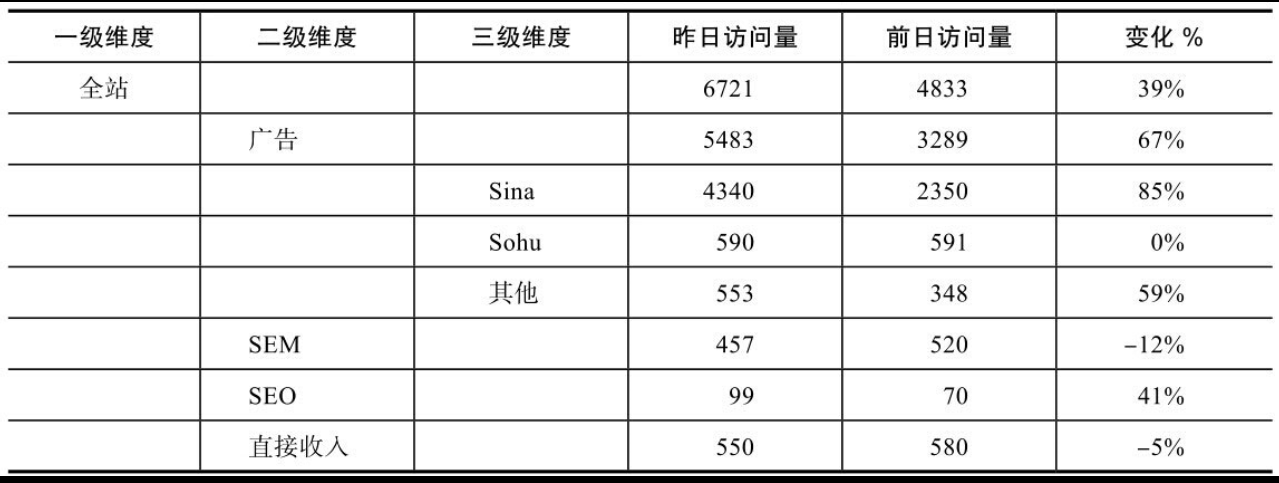## 案例背景

• 二级渠道以SEM为基准包括百度、谷歌、360等；
• 三级渠道以百度为基准包括关键字、网盟等；
• 四级渠道以关键字为基准包括不同的广告计划；
• 五级渠道以广告计划为基准可以细分到不同的广告组；
• 六级渠道以广告组为基础可以细分到不同的关键字。

1. 统计全站在一定周期内、特定指标下的数据环比变化量和环比变化率。
2. 指定要分析日期并获得该日期及其前1天的数据。
3. 以全站数据为基准，下探第一层级维度并对指定日期和其前1天的数据做分类汇总。
4. 计算第一层级维度下分类汇总后的两天数据的差值并得到环比变化量和环比变化率。
5. 对第一层级维度下的变化量排序，并分别获得环比变化量最大和最小情况下的维度名称、变化量和变化率。
6. 计算下一层级变化量与上一层级变化量的比值，变化量最大值和最小值的比例将被定义为正向贡献率和负向贡献率。
7. 循环上述步骤，直至所有层级都计算完成。
8. 使用树形图展示所有层级下的变化量最大和最小的维度信息包括维度名称、环比变化量、环比变化率、贡献率等信息。

## 案例数据

• date：日期，格式是YYYY/MM/DD。
• source：流量来源一级分类，来源于业务部门的定义。
• site：流量来源二级分类，来源于业务部门的定义。
• channel：流量来源三级分类，来源于业务部门的定义。
• media：流量来源四级分类，来源于业务部门的定义。
• visit：访问量。

## python代码

import datetime
import pandas as pd
import numpy as np
from graphviz import Digraph  # 画图用库
# 载入数据
# 显示部分数据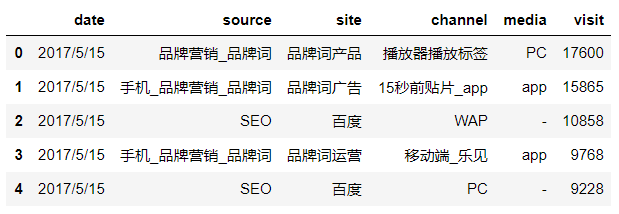# 数据类型
df.dtypes

‘‘’
date       object
source     object
site       object
channel    object
media      object
visit      object
dtype: object
‘’’

# 查看缺失值
df.isna().sum()

‘’‘
date       0
source     0
site       0
channel    0
media      0
visit      0
dtype: int64
’‘’


• 将date列转换为日期型，便于后期基于数据做筛选以及日期计算。
• 将visit列中的“-”进行转换。由于从网站分析工具导出时，“-”代表的是没有数据，因此这里转换为0。
# date列数据转化为日期型数据
df['date'] = df.date.astype('datetime64[ns]')
# 将visit列中的“-”转换为0
df['visit'] = df.visit.replace('-', 0).astype('int64')


# 获得每天visit的汇总
day_summary = df.iloc[:,-1].groupby(df.date).sum()
# 通过差分求平移一天后的变化
day_change_value = day_summary.diff(1).rename('change')
# 求变化率
day_change_rate = (day_change_value / day_summary).round(3).rename('change_rate')
# 合并
df_visit = pd.concat((day_summary, day_change_value, day_change_rate), axis=1)
# 展示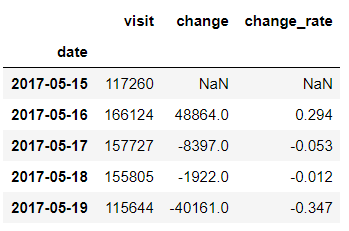# 趋势观察
df_visit.visit.plot()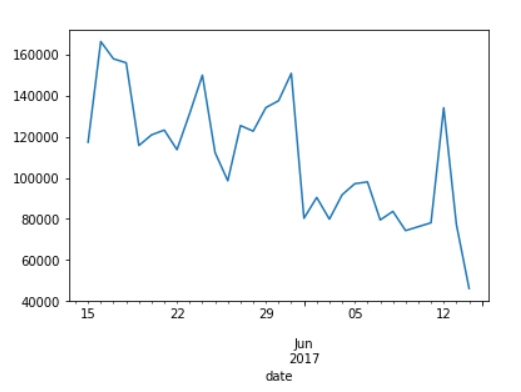# 指定要分析的日期
the_day = pd.datetime(2017, 6, 7)
# 获取前一天日期
previous_day = the_day - datetime.timedelta(1)
# 获取指定日期数据
the_day_tmp = df[df.date == the_day].rename(columns={'visit': the_day})
# 获取前一天数据
previous_day_tmp = df[df.date == previous_day].rename(columns={'visit': previous_day})
# 指定需要分析4个维度
dimension_list = df.columns.tolist()[1:-1]
# 每层分裂节点名称列表
split_node_list = ['全站']
# 每层分裂节点对应的总变化量
change_list = []
# 每层最大增长贡献最大的1个维度
increase_node_list = []
# 每层最小增长贡献最大的一个维度
decrease_node_list = []

# 遍历每一个维度
for dimension in dimension_list:
# 获取指定日期的特定维度和访问量
the_day_merge = the_day_tmp[[dimension, the_day]]
# 获取前一天的特定维度和访问量
previous_day_merge = previous_day_tmp[[dimension, previous_day]]

# 对指定日期的特定维度汇总
the_day_groupby = the_day_merge.groupby(dimension).sum()
# 对前一天的维度汇总
previous_day_groupby = previous_day_merge.groupby(dimension).sum()

# 讲两天的数据合并
merge_data = the_day_groupby.join(previous_day_groupby, how='outer')

# 将缺失值替换为0
merge_data = merge_data.fillna(0)

# 计算环比变化量
merge_data['change'] = merge_data[the_day] - merge_data[previous_day]

# 计算环比变化率
merge_data['change_rate']  =  merge_data['change']  /  merge_data[previous_day]

# 获取分裂节点的总变化值
total_change = merge_data.change.sum()

# 将变化值加入列表
change_list.append(total_change)

# 安环比变化量正向排序
merge_data.sort_values('change', inplace=True)

# 获得增长变化量最大节点名称
max_increase_node = merge_data.index[-1]

# 获得最大值节点变化量以及变化比例
max_value, max_rate = merge_data.loc[max_increase_node][2:4]

# 将最大值信息追加到列表
increase_node_list.append([max_increase_node,  int(max_value),  max_rate])
# 获得增长变化量最小值节点名称
min_increase_node = merge_data.index
# 获得最小值节点变化量以及变化比例
min_value, min_rate = merge_data.loc[min_increase_node][2:4]
# 将最小值信息追加到列表
decrease_node_list.append([min_increase_node,  int(min_value),  min_rate])

# 判断增长方向
if total_change >= 0:
# 将分裂节点定义为增长最大值节点
split_node_list.append(max_increase_node)
# 通过分裂节点的个数判断所处分裂层级
rules_len = len(split_node_list)
# 按照相应的维度过滤出指定日期符合最大节点条件的数据
the_day_tmp = the_day_tmp[the_day_tmp[dimension_list[rules_len-2]] == max_increase_node]
# 按照相应的维度过滤出前一天符合最大节点条件的数据
previous_day_tmp = previous_day_tmp[previous_day_tmp[dimension_list[rules_len-2]] == max_increase_node]
else:
split_node_list.append(min_increase_node)
# 通过分裂节点的个数判断所处分裂层级
rules_len = len(split_node_list)
# 按照相应的维度过滤出指定日期符合最大节点条件的数据
the_day_tmp = the_day_tmp[the_day_tmp[dimension_list[rules_len-2]] == min_increase_node]
# 按照相应的维度过滤出前一天符合最大节点条件的数据
previous_day_tmp = previous_day_tmp[previous_day_tmp[dimension_list[rules_len-2]] == min_increase_node]


#### 作图 ####
node_style = {'fontname': "SimSun", 'shape': 'box'}  # 定义node节点样式
edge_style = {'fontname': "SimHei", 'fontsize': '11'}  # 定义edge节点样式
top_node_style = '<<table><tr><td bgcolor="black"><font color="white">{0}</font></td></tr><tr><td>环比变化量:{1:d}</td></tr><tr><td>环比变化率:{2:.0%}</td></tr></table>>'  # 定义顶部node节点标签样式
left_node_style = '<<table><tr><td bgcolor="chartreuse"><font color="black">{0}</font></td></tr><tr><td>环比变化量:{1}</td></tr><tr><td>环比变化率:{2:.0%}</td></tr></table>>'  # 定义左侧node节点标签样式
right_node_style = '<<table><tr><td bgcolor="lightblue"><font color="black">{0}</font></td></tr><tr><td>环比变化量:{1}</td></tr><tr><td>环比变化率:{2:.0%}</td></tr></table>>'  # 定义右侧node节点标签样式
dot = Digraph(format='png', node_attr=node_style, edge_attr=edge_style)  # 创建有向图

for i in range(4):  # 循环读取每一层
node_name = split_node_list[i]  # 获得分裂节点名称
node_left, max_value, max_rate = increase_node_list[i]  # 获得增长最大值名称、变化量和变化率
node_right, min_value, min_rate = decrease_node_list[i]  # 获得增长最小值名称、变化量和变化率
node_change = change_list[i]  # 获得分裂节点的总变化量-非分裂节点变化量
node_label_left = left_node_style.format(node_left, max_value, max_rate)  # 左侧节点显示的信息
node_label_right = right_node_style.format(node_right, min_value, min_rate)  # 右侧节点显示的信息
if i == 0:  # 如果是顶部节点，则单独增加顶部节点信息
day_data = df_visit[df_visit.index == the_day]  # 获得顶部节点的数据
former_data = day_data.ix[0, 1]  # 获得全站总变化量
node_lable = top_node_style.format(node_name, int(former_data), day_data.ix[0, 2])  # 分别获取顶部节点名称、变化量和变化率
dot.node(node_name, label=node_lable)  # 增加顶部节点
contribution_rate_1 = float(max_value) / former_data  # 获得左侧变化量贡献率
contribution_rate_2 = float(min_value) / former_data  # 获得右侧变化量贡献率
if node_change >= 0:  # 如果为增长，则左侧为正向
edge_lablel_left = '正向贡献率:{0:.0%}'.format(contribution_rate_1)  # 左侧边的标签信息
edge_lablel_right = '反向贡献率:{0:.0%}'.format(contribution_rate_2)  # 右侧边的标签信息
former_data = max_value  # 获得上一层级变化量最大值
else:  # 如果为下降，则右侧为正向
edge_lablel_left = '反向贡献率:{0:.0%}'.format(contribution_rate_1)  # 左侧边的标签信息
edge_lablel_right = '正向贡献率:{0:.0%}'.format(contribution_rate_2)  # 右侧边的标签信息
former_data = min_value  # 获得上一层级变化量最大值
dot.node(node_left, label=node_label_left)  # 增加左侧节点
dot.node(node_right, label=node_label_right)  # 增加右侧节点
dot.edge(node_name, node_left, label=edge_lablel_left, color='chartreuse')  # 增加左侧边
dot.edge(node_name, node_right, label=edge_lablel_right, color='lightblue')  # 增加右侧边

dot.view('change summary')  # 展示图形结果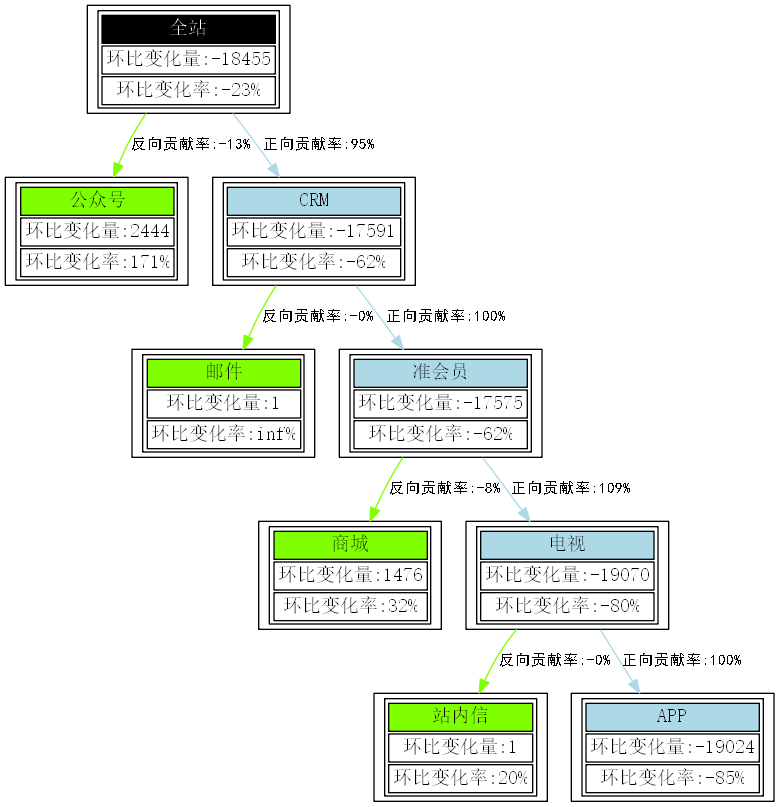## 结论

• 横向因子，即查询哪些特征对于上一层级的变化有主要的正向和负向贡献，这是贡献率的来源；
• 纵向因子，即查询每个节点本身相对前1天的环比变化率，这是其本身随着时间的变化特征，能有效了解其自身波动水平。

## 补充

• 基于网络转发的传播关系图；
• 个人关系联络图；
• 基于有时间序列的流程图；
• 网络拓扑关系图；
• 信息流和事件流图。

### 参考：

《python数据分析与数据化运营》 宋天龙07-03
03-222万+
12-195430
12-24680
05-281893
02-2282
03-191059
06-25827
12-01648
01-145067
12-051万+
12-131万+
08-187312
01-101万+
04-152万+
11-18266
04-264357